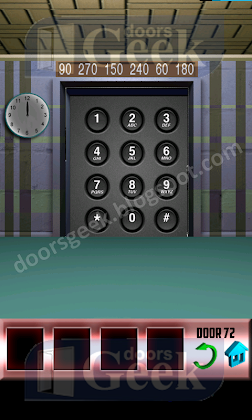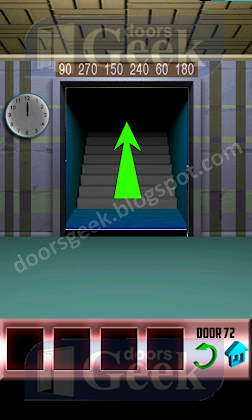## Wednesday, April 24, 2013

### 100 Doors Level 72 Walkthrough1. The clock is the starting point, and the numbers above the door are degrees. You have to calculate the degrees in the clock to get the code. We have: 12 + 90 degrees = 3 12 + 270 degrees = 9 12 + 150 degrees = 5 12 + 240 degrees = 8 12 + 60 degrees = 2 12 + 180 degrees = 6 So the code you'll need to enter is: 3958262. Now you can play the next level.